# NCERT Class 10: Science Learning Concepts

### Science: Chapter 12-Electricity-Concepts

12.2 Electric Potential and Potential Difference
Two unlike charges attract each other. Therefore, if you try to separate the two charges from each other, electric forces appear naturally. To keep them separate you have to do work on the charge, against their natural force of attraction. And according to the principle of conservation of energy, this work done is stored in the system of charges as electrostatic potential energy. Electric potential energy is present naturally whenever opposite charges have been separated or like charges have been assembled together. The amount of stored energy depends on the amount of separation of opposite charges as well as the amount of equal charges assembled together. This is similar to the gravitation analogy. You need to do work on an object of certain mass, against the force of gravity, in order to lift the object to a certain height from the surface of the earth. The work done is stored as the gravitational potential energy of the object and earth system. More the height or more the weight, more is the potential energy of the object-earth system.
Electric potential is defined as the electric potential energy per unit positive charge. We can define the electric potential difference between two points in a conductor as the energy required or the amount of work done by the electric force in moving a unit positive charge from one point to the other. By convention, a positive charge is always at a higher potential than a negative charge. Whenever there is a potential difference between two points in a conductor, charges rush down the potential difference. A positive charge moves from region of higher potential to lower potential. A negative charge has the tendency to move from a region of lower potential to higher potential. It takes energy to move the charges the other way. The voltage-altitude analogy is again helpful. Just as water rushes down with more energy from a high altitude to a horizontal level due to the pressure difference, charges rush down a steep potential difference or ‘energy slope’. In this book, we will consider the motion of positive charges unless specified otherwise. Also, it takes energy to pump water from a valley to a high altitude.
If W is the amount of work done in moving a charge Q from one point to the other, then the potential difference between the two points is:
$Potential difference = \frac{work done}{charge}$V = \frac{W}{Q}$The SI unit of potential difference is$\frac{joules}{coulomb}$or volts(denoted by symbol V) in honour of Italian Count and inventor, Alessandro Volta, who invented the first cell that produced a reliable potential difference. If 1 joule of work is done in moving a charge of 1 coulomb from one point to the other, then the potential difference between the two points is said to be 1 volt.$1 volt = \frac {1 joule}{1 coulomb}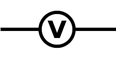A voltmeter is used to measure the potential difference between the two points of a conductor. To measure the potential difference between two points of a conductor you have to compare the energy each unit charge has at one point with the energy of the charge at the other point. Therefore you have to connect the terminals of voltmeter across the two points, or in parallel, with the conductor.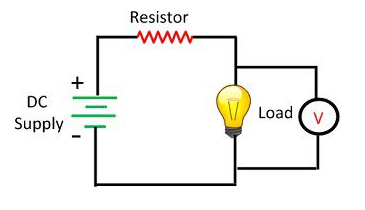The energy or ‘push’ to a charge in a conductor is provided by an electric cell which can maintain a constant potential difference between its two terminals. A cell is denoted by two lines: a thin and long line being the positive terminal and a thick and short line as the negative terminal. The positive terminal is at a higher potential with respect to the negative terminal. The potential difference (or more generally, the voltage)here is thus the amount of work that must be done on a unit positive charge, to move it from the negative plate to the positive plate, against the natural electric force that is directed from positive terminal to the negative terminal inside the cell. The energy comes from the chemical reactions occurring inside the cell. Other sources such as a generator expends the mechanical energy to generate a potential difference. The more the separation, more is the potential difference. Large potential differences can be generated by separating a large amount of equal and opposite charges. A typical cell can generate about 1.5 or 2 V. Higher voltages are accomplished with a chain of cells connected end to end (in series),which is called a battery. A torch/flashlight has two cells of 1.5 V each connected end to end. In this chain of cells, each unit positive charge gains an energy of 1.5 J each time it moves from the negative plate to the positive plate through a cell. Thus at the end of the chain, the charge has accumulated the energy of 1.5 J times 2, equals to 3 J of energy. A 9 V alkaline battery consists of six cells of 1.5 V each. Similarly, a 12 V lead-acid battery has six cells of 2 V each connected in series.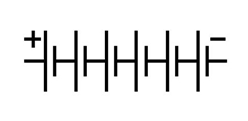Electric Current
Static electricity constitutes charges at rest. Current electricity involves flow of electric charges. In a conductor such as a metal, the conduction electrons are in random motion within the material. But, at any instant, the net flow of charge through any particular cross-section is zero.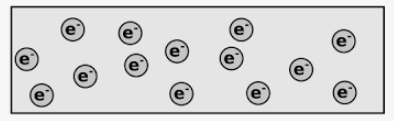Only when the ends of the conductor is connected to the two terminals of a cell, the electrons feel a push and move slowly or drift through the metal. We say that at any instant, there is a net flow of charge through a cross-section of the conductor, or electric current is flowing through the conductor. Therefore, electric current is defined as the flow of net charge through a cross-section of the material in one second. Or simply, current is the rate of flow of electric charge.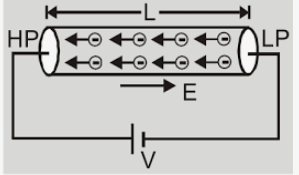If a net charge Q flows through a cross-section of a conductor in a time t, the electric current I is
Current = Amount of charge passing through a cross-section/time taken
$I =\frac{Q}{t} Electric current is measured in SI unit of amperes(denoted by symbol A) after French scientist Andre-Marie Ampere. If 1 C of charge passes through a cross-section of a conductor in 1 s, then we say that a current of 1 A flows through the conductor.$1 ampere = \frac{1 coulomb}{1 second}
An ammeter denoted by the symbol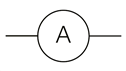is used to measure the amount of current flowing in a wire. To measure the current, you have to break the wire and insert the ammeter so that the current to be measured passes through the meter. Therefore an ammeter is connected in series with the conductor. Large currents are measured in kiloamperes $(kA, 1 kA = 10^3 A)$. Smaller currents in television circuits are measured in milliamperes (mA, 1 mA = 10^-3 A) or microamperes $\muA, 1 \mu A= 10^{-6} A$. Even smaller currents in the circuits of mobile phones or computer chips are measured in nanoamperes $(nA, 1 nA = 10^{-9} A)$or picoamperes $(pA, 1 pA = 10^{-12} A)$. The electrical nerve impulses in our body constitute very little current. A current of about 1 A generally flows through the filament of an electric bulb. A dangerously large amount of current discharges during a lightning. In our solar system, the stream of positive ions, protons and electrons emanating from the sun constitute the current of solar wind. In our galaxy, mostly the energetic protons constitute the current of cosmic rays. In this book, we shall only refer to the electric current flow in metals.
The direction of current: We know that it is actually the flow of conduction electrons through metals that constitute the electric current in wires. But unfortunately, Franklin’s choice of naming the charges made the electrons negative. By the time the mistake was recognised, it was too late to fix. And we have had to deal with current flow as the flow of imaginary positive charges in the opposite direction since then. The flow of positive charges is called conventional current. The flow of electrons is called electronic current.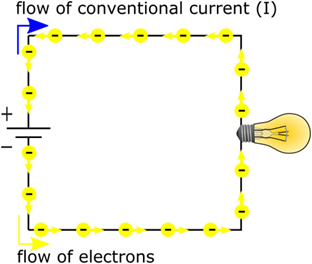The direction of conventional current is opposite to the direction of electronic current.
The end result of the flow of positive charges is same as the end result of flow of equal negative charges in opposite direction.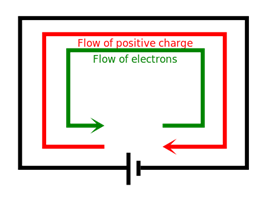We can however illustrate by an example that it does not matter which of the two charge flow constitute the current. For example, in an electric cell, if electrons from left end move to the right end, then they leave that left end with net positive charge and the right end becomes negatively charged. Similarly, if positive elementary charges drift from the right end to the left end, this leaves the right end with a net negative charge and the left end becomes positively charged. So the end results of movement of both the charges is same. The flow of negative charges has the same result as the flow of positive charges in the opposite direction. In this book, however, we shall always refer current as the conventional current unless specified otherwise.